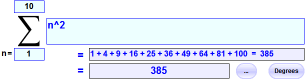Sigma Notation

Σ   This symbol (called Sigma) means "sum up"

I love Sigma, it is fun to use, and can do many clever things.

So Σ means to sum things up ...

Sum What?

Sum whatever is after the Sigma:

Σ
n
so we sum n

But What Values of n ?

The values are shown below
and above the Sigma:

4
Σ
n=1
n
it says n goes from 1 to 4,
which is 1, 2, 3 and 4

OK, Let's Go ...

So now we add up 1,2,3 and 4:

4
Σ
n=1
n = 1 + 2 + 3 + 4 = 10

Here it is in one diagram:More Powerful

But Σ can do more powerful things than that!

We can square n each time and sum the result:

4
Σ
n=1
n2 = 12 + 22 + 32 + 42 = 30

We can add up the first four terms in the sequence 2n+1:

4
Σ
n=1
(2n+1) = 3 + 5 + 7 + 9 = 24

And we can use other letters, here we use i and sum up i × (i+1), going from 1 to 3:

3
Σ
i=1
i(i+1) = 1×2 + 2×3 + 3×4 = 20

And we can start and end with any number. Here we go from 3 to 5:

5
Σ
i=3
i i + 1 = 34 + 45 + 56

There are lots more examples in the more advanced topic Partial Sums.

You can try some of your own with the Sigma Calculator.Why is it called "Sigma"Sigma is the upper case letter S in Greek. And S stands for Sum.